## Monday, January 25, 2010

### Irodov Problem 2.15

From the ideal gas law equation we know that,From Problem 2.13 we know that,From (1) and (2) we have,In (4), h-h0 represents height above sea level and P0 the atmospheric pressure at sea level and T I am guessing is assumed to be 273K.

So at a height of 5 Km above sea level we have,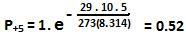Similarly at a height of 5 Km below sea level we have,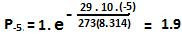### Irodov Problem 2.14

From Problem 2.13 we know that,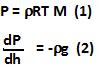From the problem definition we know that,From (2) and (3) we have,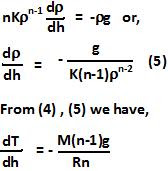### Irodov Problem 2.13From the ideal gas law equation we know that,M being the molar mass of the gas.

We also know that the pressure at any level in the gas column is given by,Since the density of the gas is not changing with height, from (1) we have,### Irodov Problem 2.12

From the ideal gas equation for one mole of gas we have,Hence we have,The extreme pressure occurs when first derivative of pressure is zero.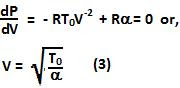The second derivative is given by,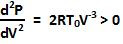Hence, the minimum pressure is given by,### Irodov Problem 2.11

Since its one mole of an ideal gas we have,a) Also since we know that,Hence we have,Extrema of the temperature will occur when the first derivative is zero.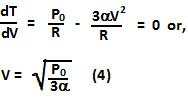The second derivative is given by,Hence this corresponds to a maximum. So we have,b) Similar to above, using ideal gas equation and given relationship between P and V the we have,Extrema of the temperature will occur when the first derivative is zero.The second derivative is given by,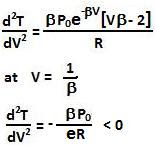Hence, this corresponds to a maximum. So from (5) and (6) we have,